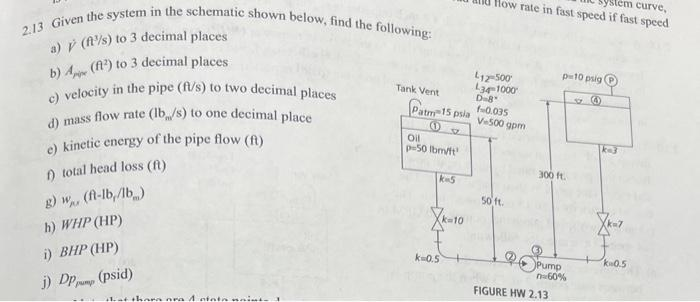# (Solved): 2.13 Given the system in the schematic shown below, find the following: a) V(f3/s) to 3 decimal p ...2.13 Given the system in the schematic shown below, find the following: a) to 3 decimal places b) to 3 decimal places c) velocity in the pipe to two decimal places d) mass flow rate to one decimal place e) kinetic energy of the pipe flow (ft) f) total head loss ( ) g) h) WHP (HP) i) j)

We have an Answer from Expert